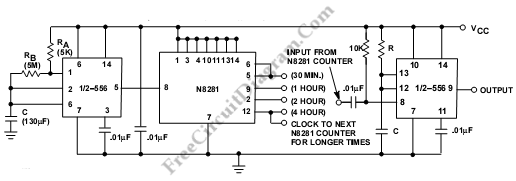# Long Time Delays with 555 TimerThis is a Long Time Delays using 555 Timer. In 555 Timer, a function of charging rate of the external capacitor is the timing. This circuit needs expensive capacitors with extremely low leakage. Practically, the components limit the time between pulse to around twenty minutes. To get longer time periods, we can connect both halves in tandem with a “divide-by” network in between. Here is the circuit:The first timer section is operated in an oscillatory mode. The periods of the first timer is 1/Fo. Then the “divide-by-N” network recieves this signal and will generate the output signal with period of N/Fo. The second half of the 555 is triggered by the output signal of “divide-by-N”. So, the total time is now a function of Fo and N. [Source: Philips Semiconductor Application Note]